# Lecture 23: Graphs and Traversals

• Tree Traversals
• Graphs
• Depth First Search

## Trees and Traversals

### Tree Definition

A tree consists of:

• A set of nodes.
• A set of edges that connect those nodes.
• Constraint: There is exactly one path between any two nodes.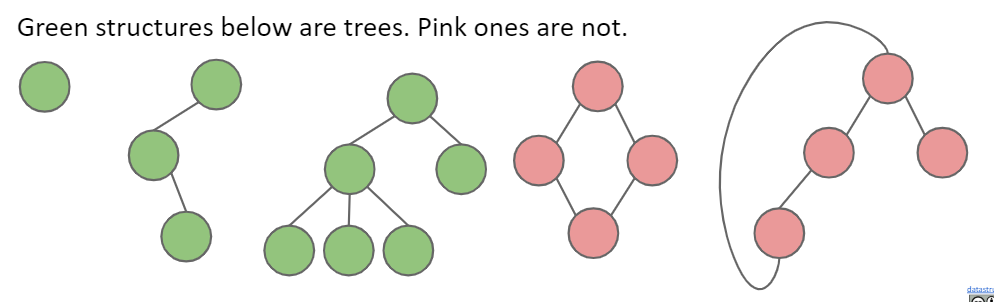### Rooted Trees Definition

A rooted tree is a tree where we’ve chosen one node as the “root”.

• Every node N except the root has exactly one parent, defined as the first node on the path from N to the root.
• A node with no child is called a leaf.

### Tree Traversal Orderings

#### Depth First Traversals

• 3 types: Preorder, Inorder, Postorder
• Basic (rough) idea: Traverse “deep nodes” (e.g. A) before shallow ones (e.g. F).
• Note: Traversing a node is different than “visiting” a node. See next slide.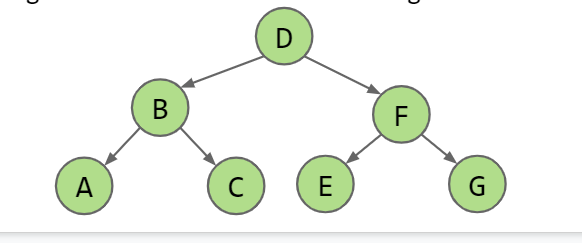#### Preorder: “Visit” a node, then traverse its children: D B A C F E G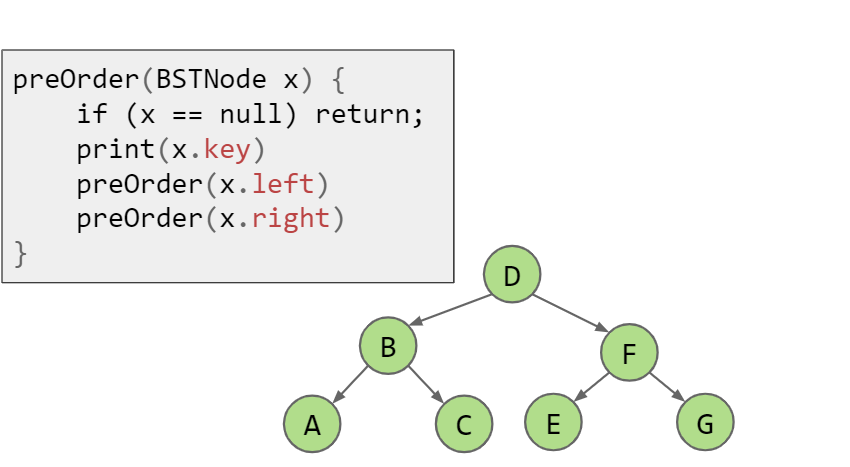#### Inorder traversal: Traverse left child, visit, then traverse right child: A B C D E F G

inOrder(BSTNode x) {
if (x == null) return;
inOrder(x.left)
print(x.key)
inOrder(x.right)
}


#### Postorder traversal: Traverse left, traverse right, then visit: ACBEGFD

postOrder(BSTNode x) {
if (x == null) return;
postOrder(x.left)
postOrder(x.right)
print(x.key)
}


#### A Useful Visual Trick (for Humans, Not Algorithms)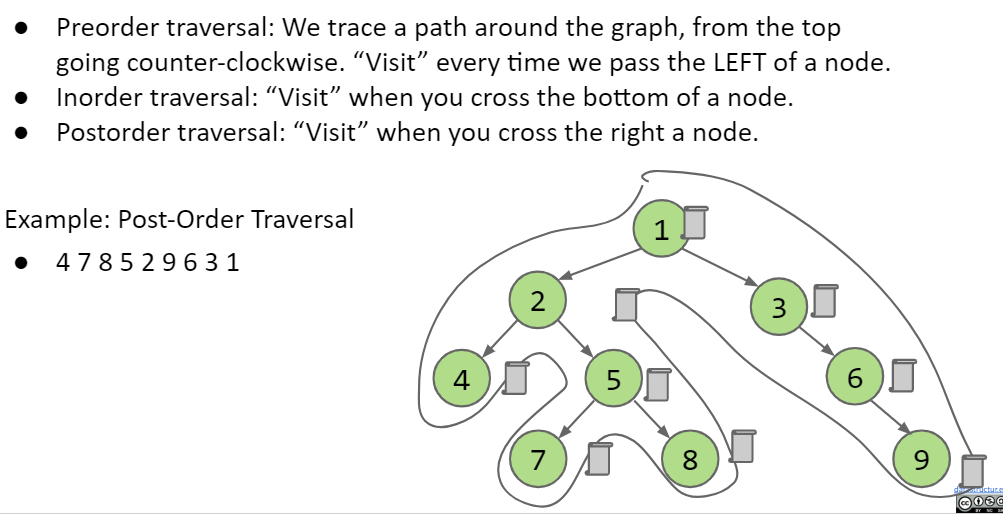#### What Good Are All These Traversals?

Example 1: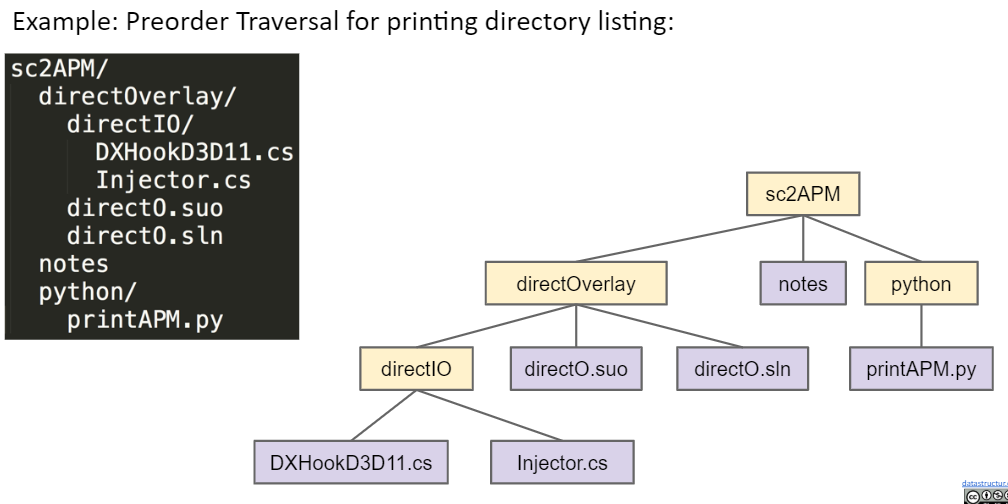Example 2: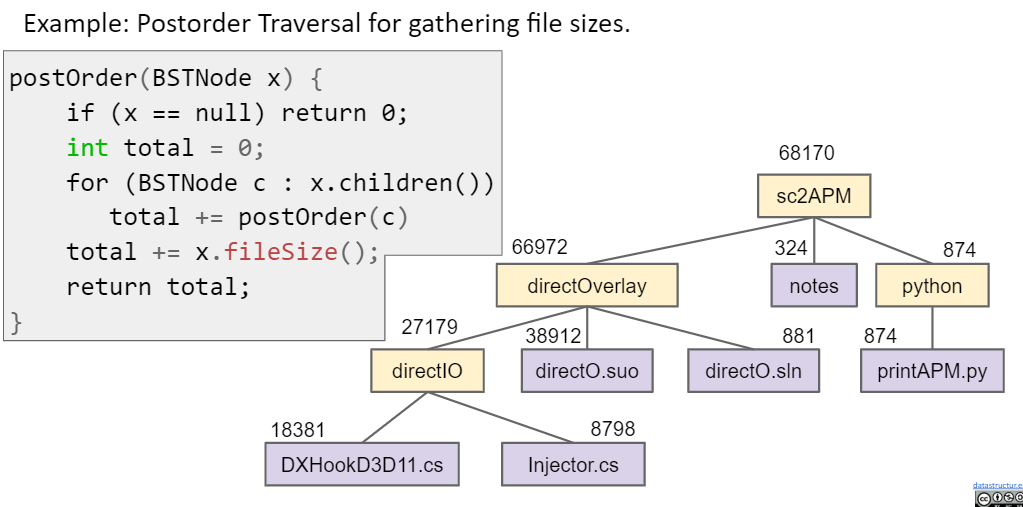## Graphs

### Graph Definition

A graph consists of:

• A set of nodes.
• A set of zero or more edges, each of which connects two nodes.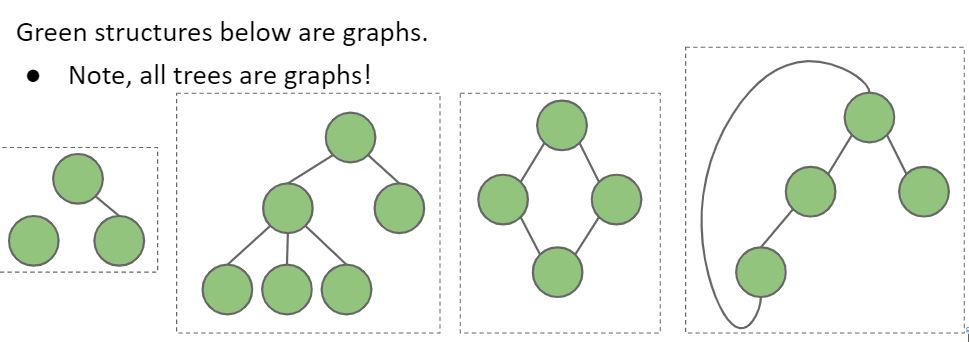A simple graph is a graph with:

• No edges that connect a vertex to itself, i.e. no “loops”.
• No two edges that connect the same vertices, i.e. no “parallel edges”.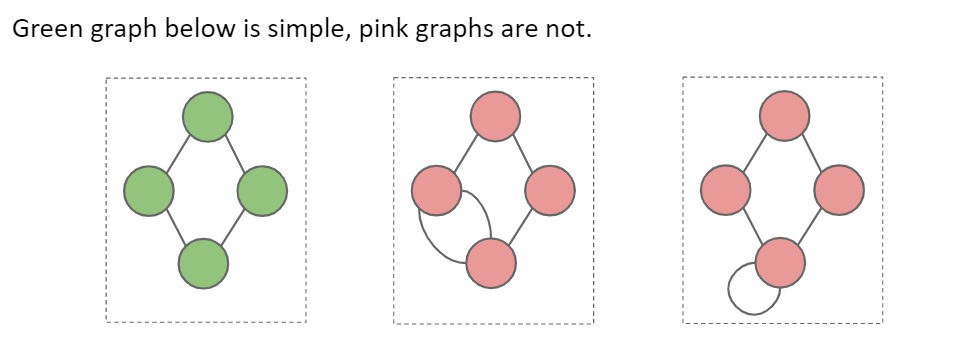In 61B, unless otherwise explicitly stated, all graphs will be simple

### Graph Types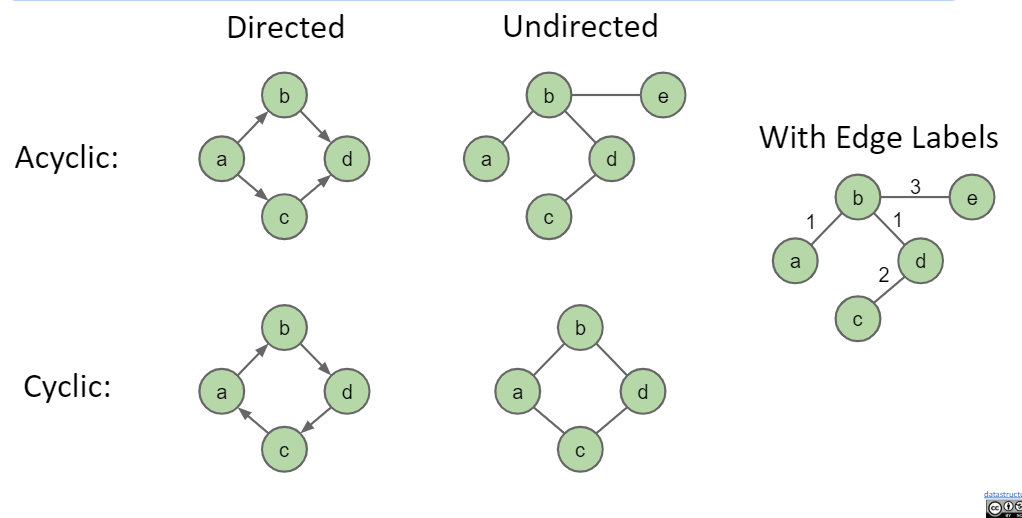### Graph Terminology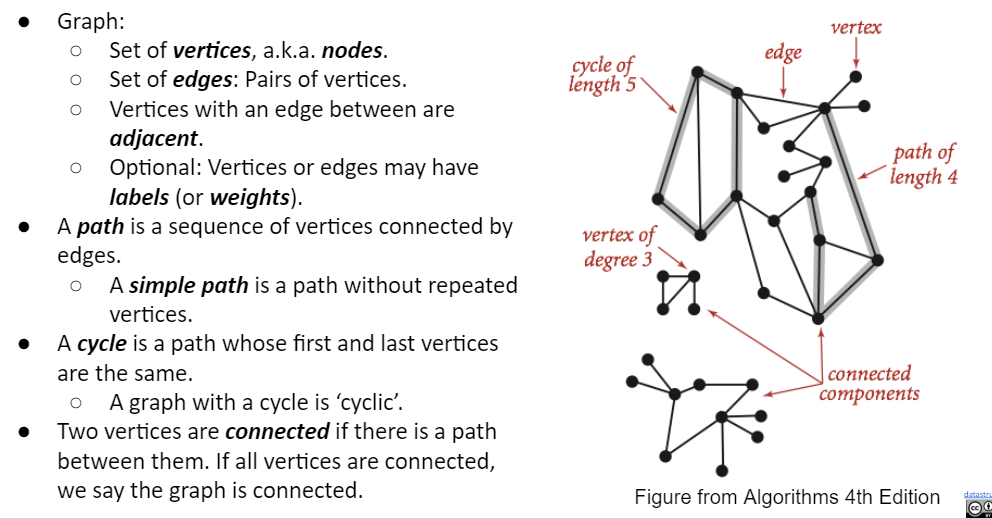## Graph Problems

### Graph Queries More Theoretically

Some well known graph problems and their common names:

• s-t Path. Is there a path between vertices s and t?
• Connectivity. Is the graph connected, i.e. is there a path between all vertices?
• Biconnectivity. Is there a vertex whose removal disconnects the graph?
• Shortest s-t Path. What is the shortest path between vertices s and t?
• Cycle Detection. Does the graph contain any cycles?
• Euler Tour. Is there a cycle that uses every edge exactly once?
• Hamilton Tour. Is there a cycle that uses every vertex exactly once?
• Planarity. Can you draw the graph on paper with no crossing edges?
• Isomorphism. Are two graphs isomorphic (the same graph in disguise)?

## Depth-First Traversal

### s-t Connectivity solved by DFS

#### s-t connectivity problem

• Given source vertex s and a target vertex t, is there a path between s and t?
• Requires us to traverse the graph somehow.
Try to come up with an algorithm for connected(s, t).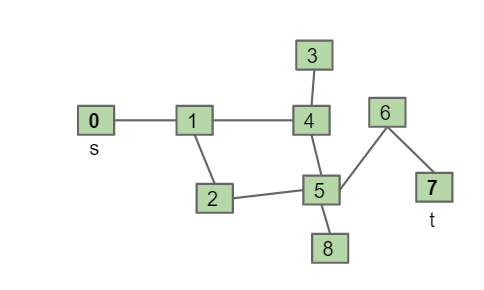##### idea

One possible recursive algorithm for connected(s, t).

• Mark s.
• Does s == t? If so, return true.
• Otherwise, if connected(v, t) for any unmarked neighbor v of s, return true.
• Return false.

demo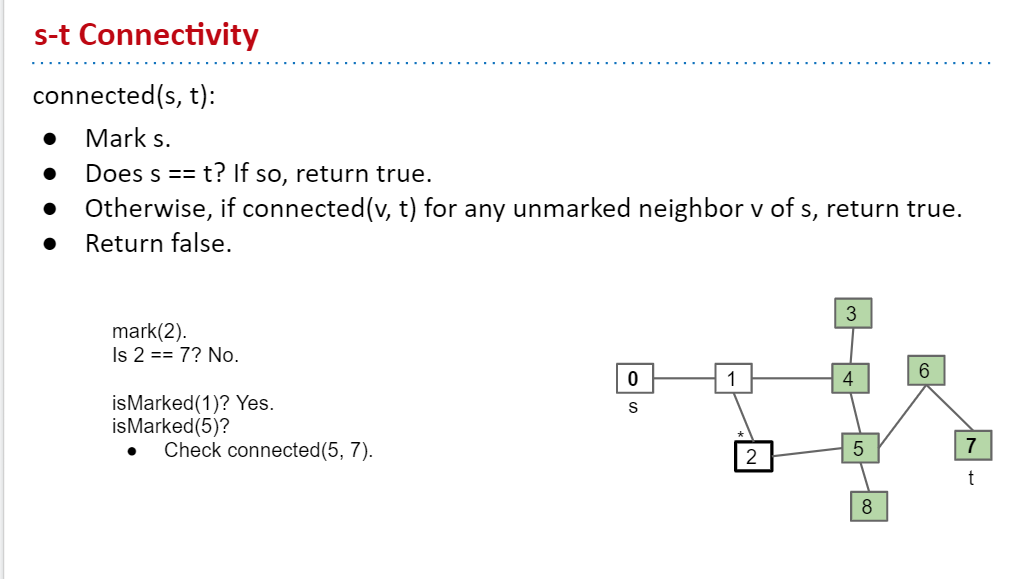### Depth First Traversal

This idea of exploring a neighbor’s entire subgraph before moving on to the next neighbor is known as Depth First Traversal.

• Example: Explore 1’s subgraph completely before using the edge 0-3.
• Called “depth first” because you go as deep as possible.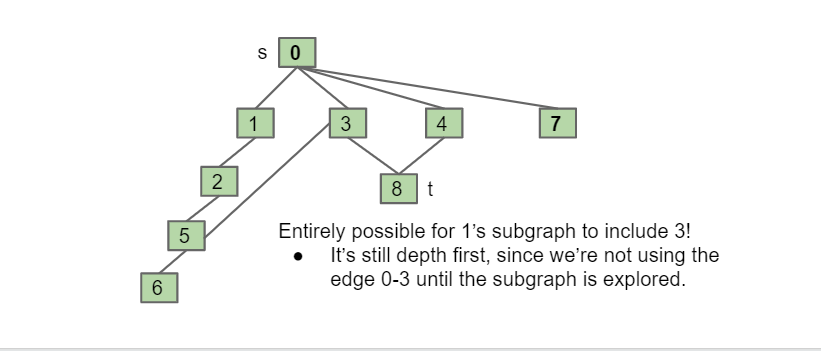Another example:

• Let’s discuss an algorithm that computes a path to every vertex.
• Let’s call this algorithm DepthFirstPaths.
• Demo: DepthFirstPaths.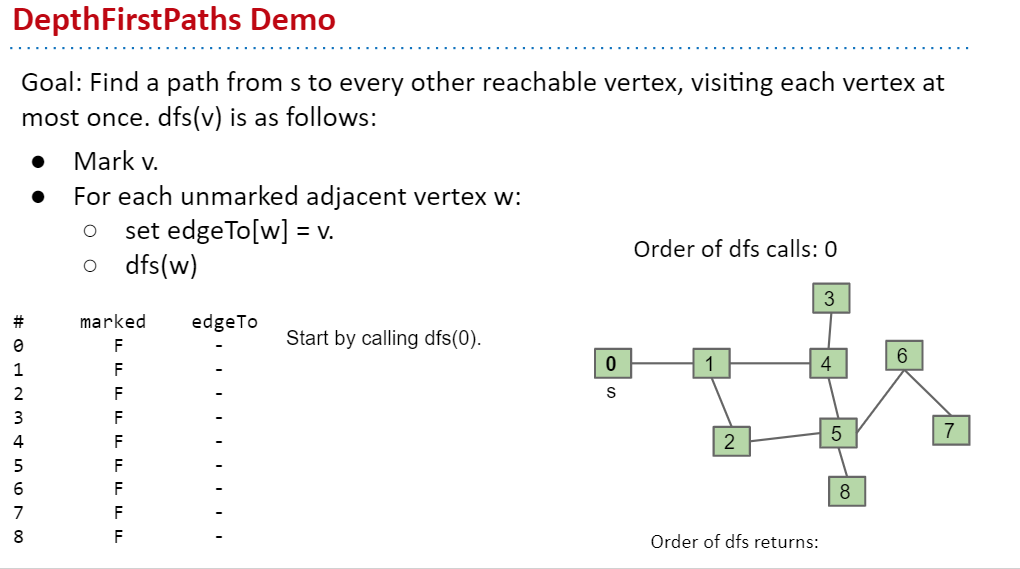## Tree Vs. Graph Traversals

### DFS Preorder

What we just did in DepthFirstPaths is called “DFS Preorder

• DFS Preorder: Action is before DFS calls to neighbors.
• Our action was setting edgeTo.
• Example: edgeTo was set before DFS calls to neighbors 2 and 4.
• One valid DFS preorder for this graph: 012543678
• Equivalent to the order of dfs calls.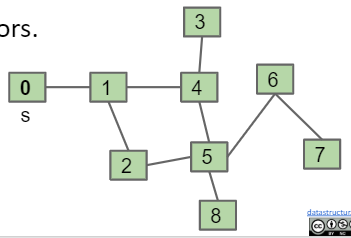### DFS Postorder.

DFS Postorder: Action is after DFS calls to neighbors.

• Example: dfs(s):

• mark(s)
• For each unmarked neighbor n of s, dfs(n)
• print(s)
• Results for dfs(0) would be: 347685210

• Equivalent to the order of dfs returns.(和DFS 执行完返回的顺序一致，即逆序)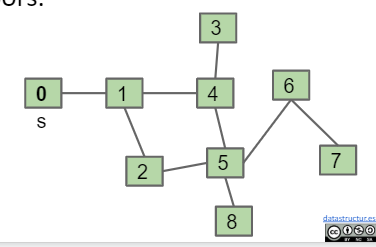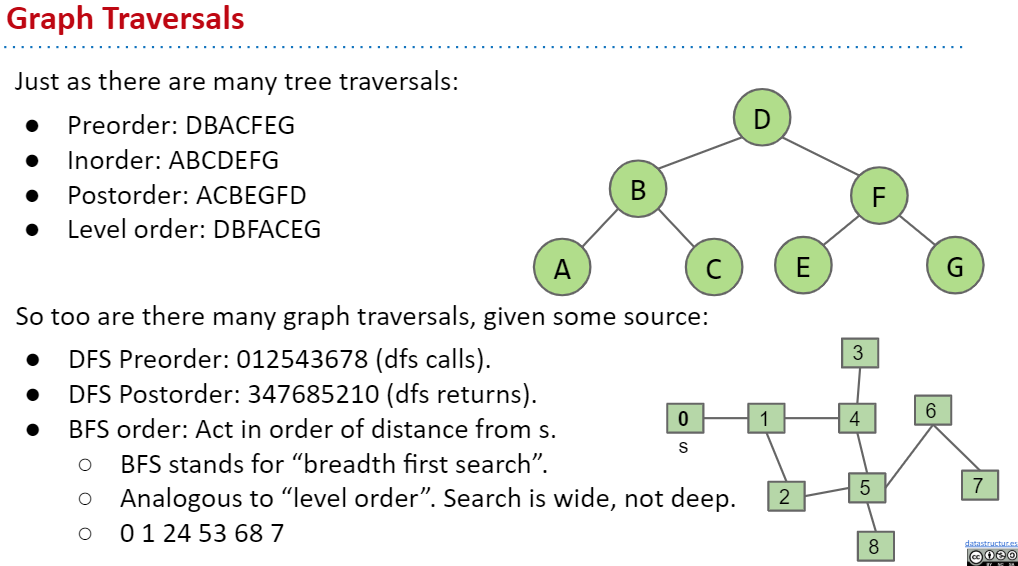## Summary

Graphs are a more general idea than a tree.

• A tree is a graph where there are no cycles and every vertex is connected.
• Key graph terms: Directed, Undirected, Cyclic, Acyclic, Path, Cycle.

Graph problems vary widely in difficulty.

• Common tool for solving almost all graph problems is traversal.
• A traversal is an order in which you visit / act upon vertices.
• Tree traversals:
• Preorder, inorder, postorder, level order.
• Graph traversals:
• DFS preorder, DFS postorder, BFS.
• By performing actions / setting instance variables during a graph (or tree) traversal, you can solve problems like s-t connectivity or path finding.©️2019 CSDN 皮肤主题: 1024 设计师: 上身试试